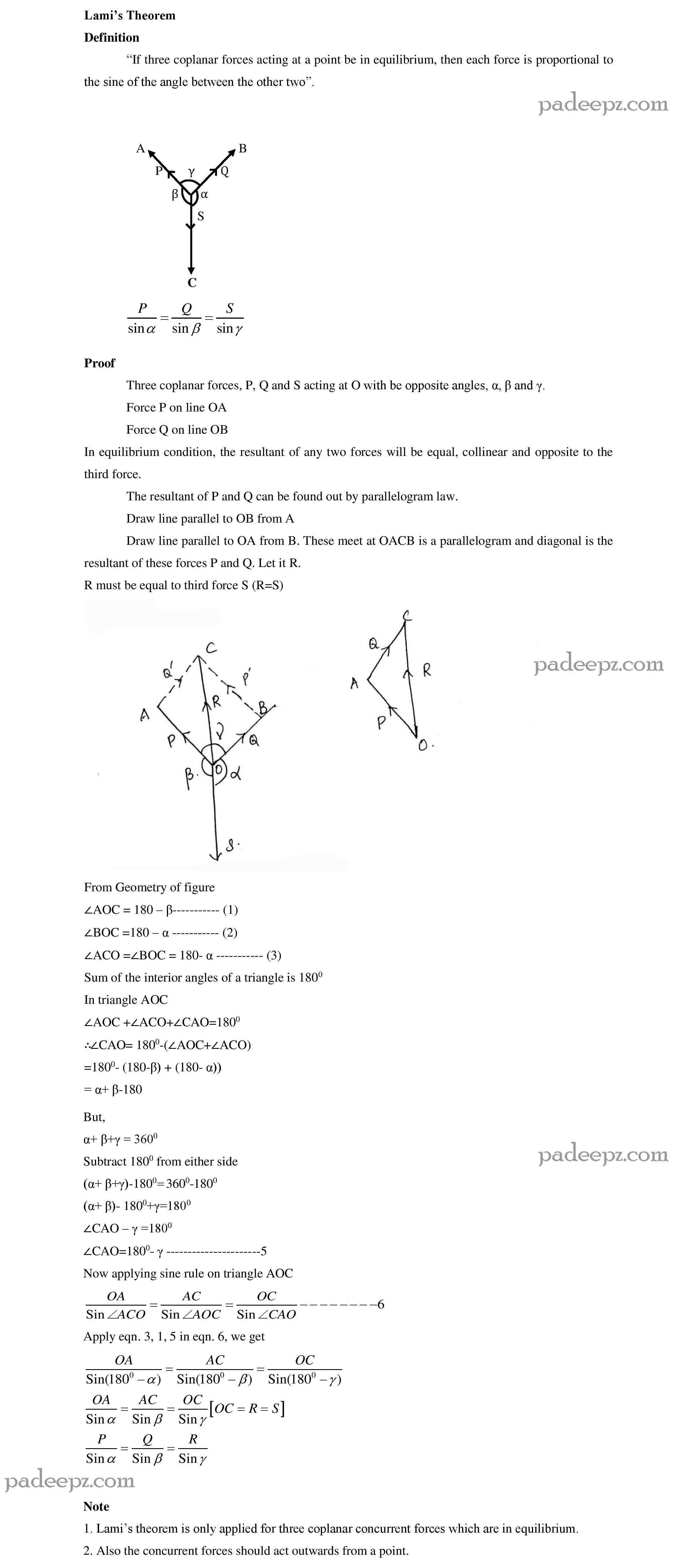Categories

# GE8292 Syllabus ENGINEERING MECHANICS EM

## UNIT I STATICS OF PARTICLES Ge8292 Syllabus Engineering mechanics EM

Introduction – Units and Dimensions – Laws of Mechanics – Lami’s theorem, Parallelogram and triangular Law of forces – Vectorial representation of forces – Vector operations of forces – additions, subtraction, dot product, cross product – Coplanar Forces – rectangular components – Equilibrium of a particle – Forces in space – Equilibrium of a particle in space – Equivalent systems of forces – Principle of transmissibility .

### UNIT II EQUILIBRIUM OF RIGID BODIES Ge8292 Syllabus Engineering mechanics EM

Free body diagram – Types of supports –Action and reaction forces –stable equilibrium – Moments and Couples – Moment of a force about a point and about an axis – Vectorial representation of moments and couples – Scalar components of a moment – Varignon’s theorem – Single equivalent force -Equilibrium of Rigid bodies in two dimensions – Equilibrium of Rigid bodies in three dimensions

#### UNIT III PROPERTIES OF SURFACES AND SOLIDS Ge8292 Syllabus Engineering mechanics EM

Centroids and centre of mass – Centroids of lines and areas – Rectangular, circular, triangular areas by integration – T section, I section, – Angle section, Hollow section by using standard formula –Theorems of Pappus – Area moments of inertia of plane areas – Rectangular, circular, triangular areas by integration – T section, I section, Angle section, Hollow section by using standard formula – Parallel axis theorem and perpendicular axis theorem – Principal moments of
inertia of plane areas – Principal axes of inertia-Mass moment of inertia –mass moment of inertia for prismatic, cylindrical and spherical solids from first principle – Relation to area moments of inertia.

##### UNIT IV DYNAMICS OF PARTICLES Ge8292 Syllabus Engineering mechanics EM

Displacements, Velocity and acceleration, their relationship – Relative motion – Curvilinear motion – Newton’s laws of motion – Work Energy Equation– Impulse and Momentum – Impact of elastic bodies.

###### UNIT V FRICTION AND RIGID BODY DYNAMICS Ge8292 Syllabus Engineering mechanics EM

Friction force – Laws of sliding friction – equilibrium analysis of simple systems with sliding friction–wedge friction-. Rolling resistance -Translation and Rotation of Rigid Bodies – Velocity and acceleration – General Plane motion of simple rigid bodies such as cylinder, disc/wheel and sphere.

Ge8292 Syllabus Engineering mechanics has been revised. Anna University in the academic year 2017-2018 has revised the syllabus.The new syllabus of engineering mechanics aims to develop capacity to predict the effect of force and motion in the course of carrying out the design functions of engineering. The university aims that the students will develop certain quality due to this revised syllabus. They are illustrate the vectorial and scalar representation of forces and moments. Analyse the rigid body in equilibrium. Determine the friction and the effects by the laws of friction. Calculate dynamic forces exerted in rigid body. Evaluate the properties of surfaces and solids.

 Subject name ENGINEERING MECHANICS Semester 2 Regulation 2017 regulation File details Syllabus in PDF format in attached

Departments that involves Engg mechanics in the second semester are

Industrial Engineering and Management, Materials Science and Engineering, Manufacturing Engineering, Mechanical and Automation Engineering, Aeronautical Engineering, Industrial Engineering, Mechanical Engineering (Sandwich), Robotics and Automation, Civil Engineering, Environmental Engineering, Agriculture Engineering, Automobile Engineering, Mechanical Engineering (MECH), Production Engineering, Mechatronics Engineering.

Search by student:

• GE8292 anna university syllabus 2017 regulation.
• 1st year EM syllabus for 2017 regulation.
• Anna university syllabus for GE8292.
• Sem 2 EM 2017 regulation.
• ENGINEERING MECHANICS syllabus R2017.
• Semester two GE8292 syllabus free.

GE8292 Important questions Engineering mechanics

GE8292 Notes Engineering mechanics EM## 1 reply on “Ge8292 Syllabus Engineering mechanics EM REGULATION 2017”Alex Nsays:

Model question paper for engineering mechanics enga irukku